## Thermocouple Circuit:

Thermocouple Circuit – One of the most commonly used methods of measurement of moderately high temperature is the thermocouple effect. When a pair of wires made up of different metals is joined together at one end, a temperature difference between the two ends of the wire produces a voltage between the two wires as illustrated in Fig.13.41

Temperature measurement with Thermocouple Circuit is based on the Seebeck ef­fect. A current will circulate around a loop made up of two dissimilar metal when the two junctions are at different temperatures as shown in Fig.13.42.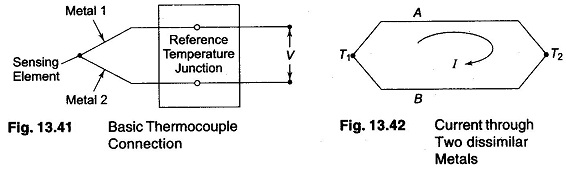When this circuit is opened, a voltage appears that is proportional to the observed seebeck current.

There are four voltage sources, their sum is the observed seebeck voltage. Each junction is a voltage source, known as Peltier emf. Furthermore, each homogenous conductor has a self induced voltage or Thomson emf.

The Thomson and Peltier emfs originate from the fact that, within conductors, the density of free charge carriers (electrons and holes) increases with temperature.

(If the temperature of one end of a conductor is raised above that of the other end, excess electrons from the hot end will diffuse to the cold end. This results in an induced voltage, the Thomson effect, that makes the hot end positive with respect to the cold end.

Conductors made up of different materials have different free-carriers densities even when at the same temperature. When two dissimilar conductors are joined, electrons will diffuse across the junction from the conductor with higher electron density. When this happens the conductor losing electrons acquire a positive voltage with respect to the other conductor. This voltage is called the Peltier emf.)

When the junction is heated a voltage is generated, this is known as seebeck effect. The seebeck voltage is linearly proportional for small changes in temperature. Various combinations of metals are used in Thermocouple’s.

The magnitude of this voltage depends on the material used for the wires and the amount of temperature difference between the joined ends and the other ends. The junction of the wires of the Thermocouple Circuit is called the sensing junction, and this junction is normally placed in or on the unit under test.

Since it is the temperature difference between the sensing junction and the other ends that is the critical factor, the other ends are either kept at a constant reference temperature, or in the case of very low cost equipment at room temperature. In the latter case, the room temperature is monitored and Thermocouple Circuit output voltage readings are corrected for any changes in it.

Because the temperature at this end of the Thermocouple Circuit wire is a reference temperature, this function is known as the reference, also called as the cold junction.

A Thermocouple Circuit, therefore consists of a pair of dissimilar metal wires joined together at one end (sensing or hot junction) and terminated at the other end (reference or cold junction), which is maintained at a known constant tempera­ture (reference temperature). When a temperature difference exists between the sensing junction and the reference junction, an emf is produced, which causes current in the circuit.

When the reference end is terminated by a meter or a recording device, the meter indication will be proportional to the temperature difference between the hot junction and the reference junction.

The magnitude of the thermal emf depends on the wire materials used and in the temperature difference between the junctions.

Figure 13.43 shows the thermal emfs for some common thermocouple ma­terials. The values shown are based on a reference temperature of 32°F.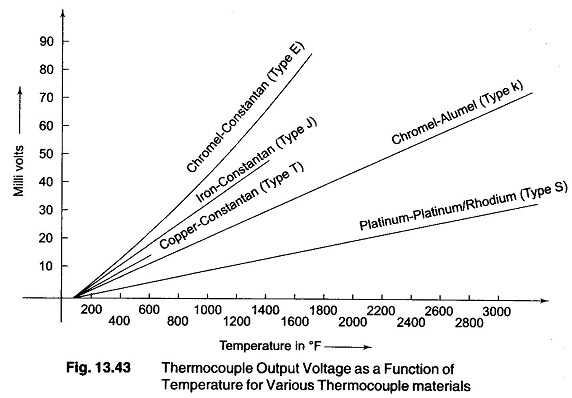The thermpcouple (TC) is a temperature transducer that develops an emf that is a function of the temperature difference between its hot and cold junctions.

A Thermocouple Circuit may be regarded as a thermometer based on thermo-emf and works on the principle that the potential between two dissimilar metals or metal alloys is a function of temperature.

Type ‘E’ Thermocouple units use Chromel alloy as the positive electrode and constantan alloy as the negative electrode.

Type ‘S’ Thermocouple produces the least output voltage but can be used over greatest temperature range.

Type ‘T’ shown in Fig. 13.44, uses copper and constantan.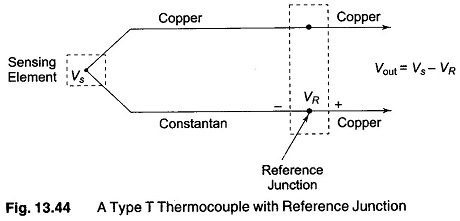Copper used, is an element and constantan used is an alloy of nickel and copper. The copper side is positive and constantan side is negative. Assuming copper wires used to connect the Thermocouple Circuit to the next stage (circuit), a second Copper-Constantan junction is (formed) produced. This junction is called as the reference junction. It generates a Seebeck voltage that opposes the voltage generated by the sensing junction. If both junctions are at the same temperature, the output voltage Vout will be zero. If the sensing junction is at a higher temperature, Vout will be proportional to the difference between the two junction temperature. The temperature cannot be derived directly from the output voltage alone. It is subjected to an error caused by the voltage produced by the reference junction. This can be overcome by placing the reference junction in an ice bath to keep it at a known temperature. This process is called as cold junction compensation as shown in Fig.13.45(a). The reference voltage is maintained at 0 °C. The reference voltage is now predictable from the calibration curve of the type ‘T’ thermocouple.

When copper is not one of Thermocouple Circuit metal then four junction circuit is formed. The type T thermocouple uses iron and constantan as the two elements shown in Fig.13.45(b). When it is connected to copper wires, two iron copper junctions result. These junctions present no additional difficulties because of the isothermal block used. This block is made of material that is a poor conductor of electricity but a good conductor of heat. Both Iron—Copper junctions will be at the same temperature and generate the same Seeback voltage and hence these two voltages will cancel. Cold junction compensation is also used as the Reference junction in this case.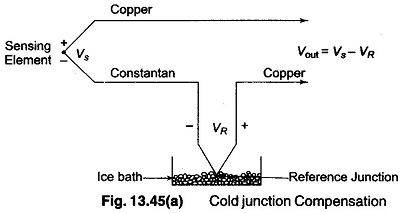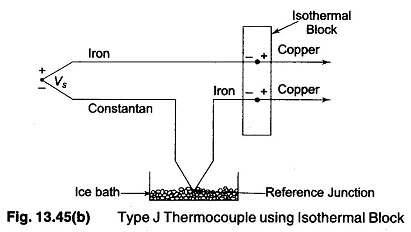The ice-baths method is not the most convenient method, to compensate the reference junction. This technique is often used in the calibration laboratory. Industry uses a different method of reference junction compensation as shown in Fig.13.46.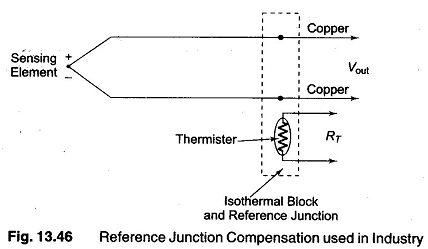The isothermal block contains two reference junctions and a thermistor. The resistance of the thermistor is a function of temperature. A circuit is used to sense this resistance and to compensate for the voltage introduced by the two reference junctions. This arrangement is sometimes called as Electronic ice point reference.

If the sensor is interfaced to a computer, the reference temperature will be converted to a reference voltage and then subtracted from the output voltage Vout This process is known as Software Compensation.

An isothermal block with one temperature sensor can provide compensation for several units.

Table 13.3 gives the construction and thermoelectric properties of various thermocouples.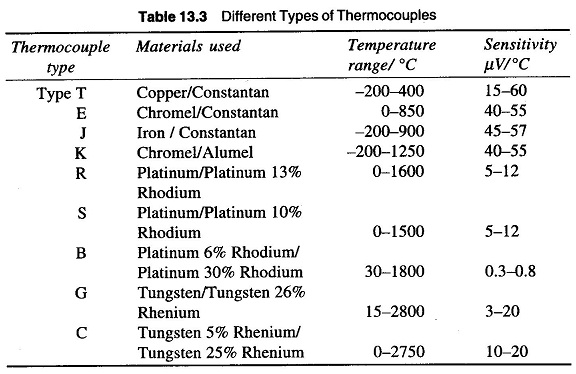For accurate measurement of hot junction temperature, the cold junction or the reference junction should be kept at 0 °C. If the reference junction is kept at the ambient temperature, then a voltage corresponding to this temperature must be added to the measurement to obtain accurate reading.

Most modern Thermocouple Circuit measurement systems employ electrical cold junction (an electronic circuit which simulates the voltage that the reference junction would generate at ambient temperature) compensation. A popular technique used for reference junction compensation used in data loggers and data acquisition systems is shown in Fig. 13.47.

The measuring junction’s terminals are screwed on an isothermal block (the temperature of which remains uniform within ± 0.05 °C). The temperature of the isothermal block is measured independently and compensating voltage is generated using electronic circuitry. This compensation voltage is combined with the emf from measuring junction to obtain the true temperature

Thermocouples are sometimes connected in series or parallel to provide increased voltage or current output.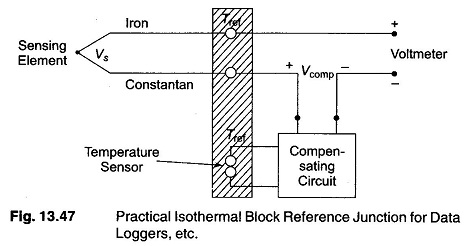In Fig.13.48(a), four thermocouples are connected in series, with wire A being positive and B being negative in each thermocouple.

The total emf between points 1 to 5 is the sum of individual thermocouple emf. An arrangement of this type is called a Thermopile and is used to obtain increased sensitivity and greater absolute emf from a thermocouple installation.

Figure 13.48(b) shows four thermocouples in parallel. This arrangement pro­vides a large current but emf is same as that of any one thermocouple.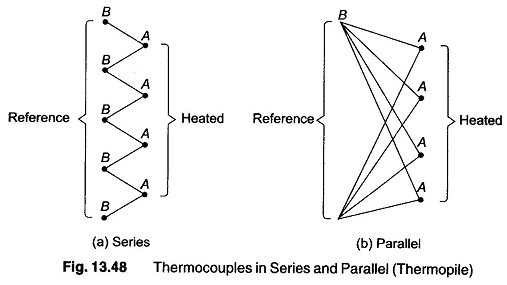Thermocouple Circuit must be protected from mechanical damage and isolated from corrosive or contaminating effect that most gases and liquids have at high temperature. The device used for this purpose are called wells or tubes depend­ing upon their physical construction or thermowells.

Thermocouples are made from a number of different metal alloys, covering a wide range of temperature from as low as —270 °C (-418 °F) to as high as 2700 °C (about 5000 °F). They may be obtained in a simple uninsulated wire form, in insulated form or inside protective sheaths or probes (sheath diameter as small as 0.25 mm).

The thermo-junction is protected from contamination from the process materials by enclosing it in a protective sheath. For example, a cupro-nickel sheath for copper/chromel thermocouple and mild sheath for iron/chromel thermocouples.

The temperature ranges covered by thermocouples make them appropriate for use in industrial furnaces as well as for measurement in the cryogenic range. Different types of thermocouples are as shown in Fig. 13.49.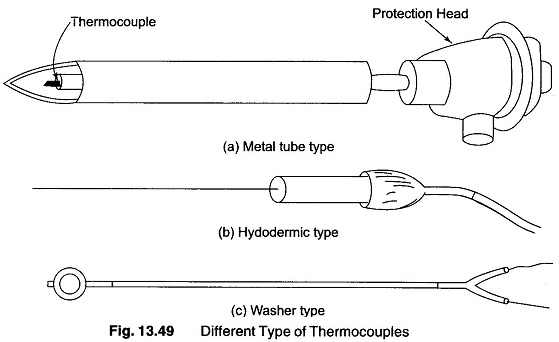1. It has rugged construction.
2. It has a temperature range from —270 °C-2700 °C.
3. Using extension leads and compensating cables, long distances transmis­sion for temperature measurement is possible.
4. Bridge circuits are not required for temperature measurement.
5. Comparatively cheaper in cost.
6. Calibration checks can be easily performed.
7. Thermocouples offer good reproducibility.
8. Speed of response is high compared to the filled system thermometer.
9. Measurement accuracy is quite good.